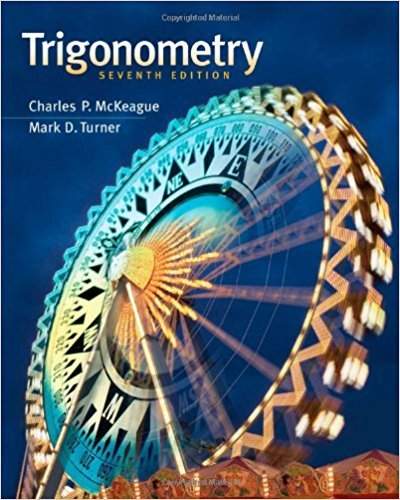×
×

# Solutions for Chapter 6.3: Trigonometric Equations Involving Multiple Angles## Full solutions for Trigonometry | 7th Edition

ISBN: 9781111826857Solutions for Chapter 6.3: Trigonometric Equations Involving Multiple Angles

Solutions for Chapter 6.3
4 5 0 241 Reviews
18
2
##### ISBN: 9781111826857

This textbook survival guide was created for the textbook: Trigonometry, edition: 7. Chapter 6.3: Trigonometric Equations Involving Multiple Angles includes 83 full step-by-step solutions. Trigonometry was written by and is associated to the ISBN: 9781111826857. This expansive textbook survival guide covers the following chapters and their solutions. Since 83 problems in chapter 6.3: Trigonometric Equations Involving Multiple Angles have been answered, more than 24586 students have viewed full step-by-step solutions from this chapter.

Key Math Terms and definitions covered in this textbook
• Back substitution.

Upper triangular systems are solved in reverse order Xn to Xl.

• Big formula for n by n determinants.

Det(A) is a sum of n! terms. For each term: Multiply one entry from each row and column of A: rows in order 1, ... , nand column order given by a permutation P. Each of the n! P 's has a + or - sign.

• Change of basis matrix M.

The old basis vectors v j are combinations L mij Wi of the new basis vectors. The coordinates of CI VI + ... + cnvn = dl wI + ... + dn Wn are related by d = M c. (For n = 2 set VI = mll WI +m21 W2, V2 = m12WI +m22w2.)

• Cofactor Cij.

Remove row i and column j; multiply the determinant by (-I)i + j •

• Condition number

cond(A) = c(A) = IIAIlIIA-III = amaxlamin. In Ax = b, the relative change Ilox III Ilx II is less than cond(A) times the relative change Ilob III lib II· Condition numbers measure the sensitivity of the output to change in the input.

• Elimination matrix = Elementary matrix Eij.

The identity matrix with an extra -eij in the i, j entry (i #- j). Then Eij A subtracts eij times row j of A from row i.

• Full row rank r = m.

Independent rows, at least one solution to Ax = b, column space is all of Rm. Full rank means full column rank or full row rank.

• Gauss-Jordan method.

Invert A by row operations on [A I] to reach [I A-I].

• Hessenberg matrix H.

Triangular matrix with one extra nonzero adjacent diagonal.

• Independent vectors VI, .. " vk.

No combination cl VI + ... + qVk = zero vector unless all ci = O. If the v's are the columns of A, the only solution to Ax = 0 is x = o.

• Iterative method.

A sequence of steps intended to approach the desired solution.

• Jordan form 1 = M- 1 AM.

If A has s independent eigenvectors, its "generalized" eigenvector matrix M gives 1 = diag(lt, ... , 1s). The block his Akh +Nk where Nk has 1 's on diagonall. Each block has one eigenvalue Ak and one eigenvector.

• Left nullspace N (AT).

Nullspace of AT = "left nullspace" of A because y T A = OT.

• Matrix multiplication AB.

The i, j entry of AB is (row i of A)·(column j of B) = L aikbkj. By columns: Column j of AB = A times column j of B. By rows: row i of A multiplies B. Columns times rows: AB = sum of (column k)(row k). All these equivalent definitions come from the rule that A B times x equals A times B x .

• Nullspace matrix N.

The columns of N are the n - r special solutions to As = O.

• Projection p = a(aTblaTa) onto the line through a.

P = aaT laTa has rank l.

• Solvable system Ax = b.

The right side b is in the column space of A.

• Special solutions to As = O.

One free variable is Si = 1, other free variables = o.

• Spectrum of A = the set of eigenvalues {A I, ... , An}.

Spectral radius = max of IAi I.

• Vector space V.

Set of vectors such that all combinations cv + d w remain within V. Eight required rules are given in Section 3.1 for scalars c, d and vectors v, w.

×